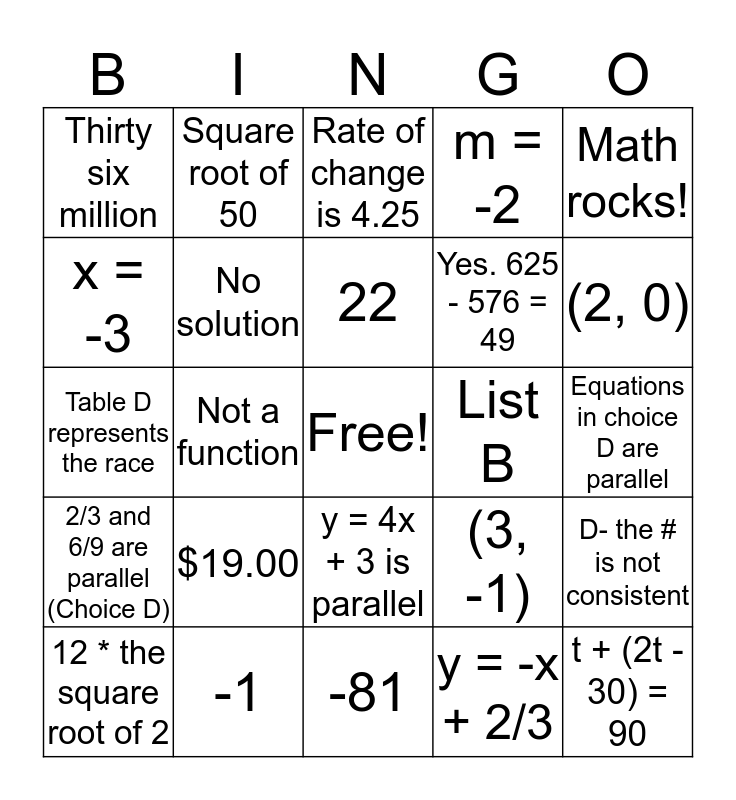# Math Review BingoThis bingo card has a free space and 24 words: \$19.00, -1, 22, 12 * the square root of 2, Math rocks!, Thirty six million, x = -3, Square root of 50, List B, y = 4x + 3 is parallel, Yes. 625 - 576 = 49, Table D represents the race, (3, -1), D- the # is not consistent, No solution, y = -x + 2/3, Equations in choice D are parallel, Rate of change is 4.25, (2, 0), Not a function, t + (2t - 30) = 90, 2/3 and 6/9 are parallel (Choice D), m = -2 and -81.

## Play Online